# Gravity Force Inside a Spherical Shell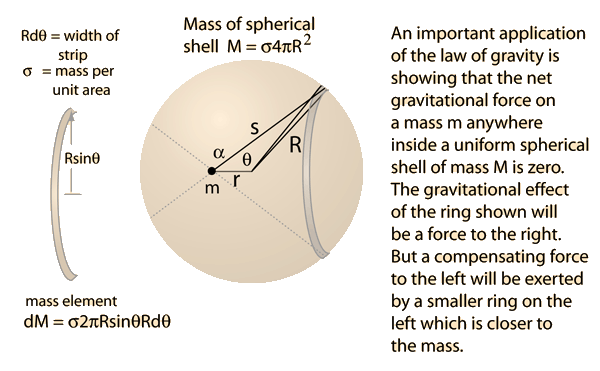For application of the law of gravity inside a uniform spherical shell of mass M, a point is chosen on the axis of a circular strip of mass. The problem is envisioned as dividing an infinitesemally thin spherical shell of density σ per unit area into circular strips of infinitesemal width. The choice of such a point involves no loss of generality because for any point inside the shell, the mass elements could be chosen so that the point is on their symmetry axis.

To set up the necessary integral, the triangle above is used to take advantage of the symmetry of the system. All components of the gravity force perpendicular to r will cancel by symmetry, and all components along r will sum. The differential element of force on mass m can be written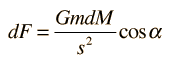where the differential element of mass dM is given by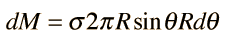The force from the entire spherical shell can be expressed as an integral over the angle θ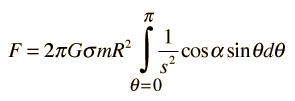To evaluate the integral, the variables s and α must be expressed in terms of the angle θ . Using the Law of Cosines with the interior angle θ givesand this can be differentiated to give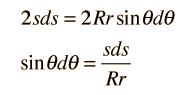Now using the Law of Cosines with the external angle α:With these relationships, we can now express the integral in terms of s instead of θ. Up to this point, the treatment is the same at that for a point outside the shell, but now the form of the limits is different. For θ = 0, s = R - r and s = r + R for θ = π.Using the area density expression σ = M/4πR2, the integral can be written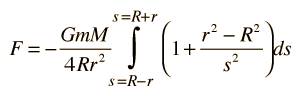Now the parts are evaluated as polynomial integrals and simplified.The net gravitational force on a point mass inside a spherical shell of mass is identically zero! Physically, this is a very important result because any spherically symmetric mass distribution outside the position of the test mass m can be build up as a series of such shells. This proves that the force from any spherically symmetric mass distribution on a mass inside its radius is zero. If a given mass m is inside a spherically symmetric distribution of mass, that part of the mass outside its radius does not contribute to the net force on it.

 Force on a mass outside a spherical shell
 Example of falling through the Earthl
Index

Newton's laws

Gravity concepts

 HyperPhysics***** Mechanics R Nave
Go Back